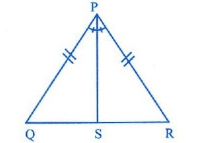Home/Class 7/Maths/

QuestionMathsClass 7

In Figure, $$PS$$ is the bisector of $$\angle P$$ and $$PQ=PR$$ .Then $$\Delta PRS$$ and $$\Delta PQS$$ are congruent by the criterion（ ）
A. $$AAA$$
B. $$SAS$$
C. $$ASA$$
D. Both (B) and (C)

D
4.64.6Solution

In $$\Delta PRS$$ and $$\Delta PQS$$
$$PQ=PR$$ [Given]
$$\angle QPS = \angle RPS$$ [Given]
$$PS =PS$$ [Common]
Hence  $$\Delta PRS \cong \Delta PQS$$ [SAS criteria]
Also, In $$\Delta PRS$$ and $$\Delta PQS$$
$$\angle PQS=\angle PRS$$ (Angles opposite to equal sides)
$$PQ=PR$$ (Given)
$$\angle QPS=\angle RPS$$ (Given)
Therefore, $$\Delta PRS \cong \Delta PQS$$ (By ASA congruency criterion)
Hence, option (D) is correct.